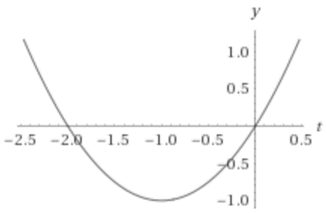# The domain and range of the function and sketch the graph.### Single Variable Calculus: Concepts...

4th Edition
James Stewart
Publisher: Cengage Learning
ISBN: 9781337687805### Single Variable Calculus: Concepts...

4th Edition
James Stewart
Publisher: Cengage Learning
ISBN: 9781337687805

#### Solutions

Chapter 1.1, Problem 37E
To determine

## To find: The domain and range of the function and sketch the graph.

Expert Solution

### Explanation of Solution

Given information:

The given function is,

f(t)=2t+t2

Consider the graph of the given function,Since the given function is defined at every point. So, the domain of the given function is all real numbers.

For the calculation of the range, consider the given equation,

f(t)=2t+t2t2+2tf(t)=0

The root of the above equation can be written as follows:

t=2±224×1×(f(t))2×1t=1±1+f(t)

The above is valid when,

1+f(t)0f(t)1

So, the range of the given function is all real numbers except f(t)1.

### Have a homework question?

Subscribe to bartleby learn! Ask subject matter experts 30 homework questions each month. Plus, you’ll have access to millions of step-by-step textbook answers!